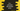# C++ program to calculate discounted price## C++ program to calculate discounted price:

In this program, we will learn how to calculate the price of an item after applying the discount. This program will take the price as an input from the user and print the discounted price.

This program will read the discount values based on predefined values.

### Discount table:

We will use the following discount table:

Amount Discount
More than 100\$ 2%
More than 500\$ 5%
More than 1000\$ 10%

So,

• If the final amount is more than 100\$, you will get a discount of 2%
• If the final amount is more than 500\$, you will get a discount of 5%
• If the final amount is more than 1000\$, you will get a discount of 10%

### Algorithm:

The program will use the below algorithm:

• Get the price as input from the user.
• Check if the price is more than any value as listed on the discount table.

• If yes, calculate the discount.
• For example, if the total price is t and if d% is the discount for that price, the user will get (d * t)/100 discount. If we subtract the discount amount from the total price, we will get the amount the user needs to pay.

### C++ program to calculate discounted price:

Let’s write down the C++ program:

``````#include <iostream>

using namespace std;

int main()
{
float totalAmount, discount = 0, amountToPaid;

cout << "Enter the total amount:" << endl;
cin >> totalAmount;

if (totalAmount > 1000)
{
discount = (10 * totalAmount) / 100;
amountToPaid = totalAmount - discount;
}
else if (totalAmount > 500)
{
discount = (5 * totalAmount) / 100;
amountToPaid = totalAmount - discount;
}
else if (totalAmount > 100)
{
discount = (2 * totalAmount) / 100;
amountToPaid = totalAmount - discount;
}
else
{
amountToPaid = totalAmount;
}

cout << "Total Amount to pay: \$" << totalAmount << endl;
cout << "Discount: \$" << discount << endl;
cout << "Amount to pay after discount: \$" << amountToPaid << endl;
return 0;
}``````

### Description:

In this program,

• totalAmount variable is initialized to hold the total amount before discount is calculated.
• discount variable is to hold the discount on the total amount and amountToPaid is the final amount to pay.
• The program asks the user to enter the total amount and stores that value in the variable totalAmount.
• It uses a if-else if-else block to check if any discount can be applied for that amount. We are using the same discount table mentioned in the beginning of this post.
• Based on the discount value, it calculates the discount and stores that value in the discount variable. It also updates the amountToPaid variable by subtracting discount from the total amount.
• If no discount can be applied, it assigns the total amount as the amount to be paid.
• At the end of the program, it prints these values.

### Sample output:

If you run this program, it will print output as like below:

``````Enter the total amount:
120
Total Amount to pay: \$120
Discount: \$2.4
Amount to pay after discount: \$117.6

Enter the total amount:
300
Total Amount to pay: \$300
Discount: \$6
Amount to pay after discount: \$294

Enter the total amount:
700
Total Amount to pay: \$700
Discount: \$35
Amount to pay after discount: \$665

Enter the total amount:
1500
Total Amount to pay: \$1500
Discount: \$150
Amount to pay after discount: \$1350

Enter the total amount:
50
Total Amount to pay: \$50
Discount: \$0
Amount to pay after discount: \$50``````

### Method 2: Another way to solve this by using a separate method:

We can also use a separate method to get the discount percentage value and the final calculation can be done at one place.

Below is the final program:

``````#include <iostream>

using namespace std;

int getDiscountPercent(int totalAmount)
{
if (totalAmount > 1000)
{
return 10;
}
else if (totalAmount > 500)
{
return 5;
}
else if (totalAmount > 100)
{
return 2;
}

return 0;
}

int main()
{
float totalAmount, discountPercent, discount = 0, amountToPaid;

cout << "Enter the total amount:" << endl;
cin >> totalAmount;

discountPercent = getDiscountPercent(totalAmount);

if (discountPercent != 0)
{
discount = (discountPercent * totalAmount) / 100;
amountToPaid = totalAmount - discount;
}
else
{
amountToPaid = totalAmount;
}

cout << "Total Amount to pay: \$" << totalAmount << endl;
cout << "Discount: \$" << discount << endl;
cout << "Amount to pay after discount: \$" << amountToPaid << endl;
return 0;
}``````
• This program is almost similar to the above one. One new variable discountPercent is introduced to keep the discount percentage.
• The getDiscountPercent method is used to get the discount percentage. It calculates the discount percentage and returns it.
• The discount percentage is calculated and checks in the if-else block. The if block checks if the discount percentage is 0 or not. If it is not 0, it calculates the discount value and the amount to be paid.

If you run this program, it will print similar output.